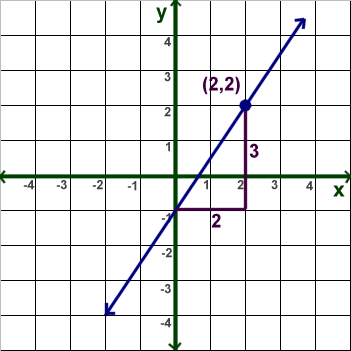# Kids Math

## Linear Equations - Slope Forms

This page assumes you have some basic knowledge of linear equations and slope. In the linear equations basics section we discussed the standard form of a linear equation where Ax + By = C.

There are other ways that linear equations can be written that can help provide useful information for graphing. They are called slope forms. There is the slope-intercept form and the point-slope form.

Slope-Intercept Form

The slope intercept form uses the following equation:

y = mx + b

In this equation, x and y are still the variables. The coefficients are m and b. These are numbers.

The advantage of putting a linear equation in this form is that the number for m equals the slope and the number for b equals the y-intercept. This makes the line the equation represents simple to graph.

m = slope
b = intercept

slope = (change in y) divided by the (change in x) = (y2 - y1)/(x2 - x1)

intercept = the point where the line crosses (or intercepts) the y-axis

Example Problems:

1) Graph the equation y = 1/2x + 1

From the equation y = mx + b we know that:

m = slope = ½
b = intercept = 11) Graph the equation y = 3x - 3

From the equation y = mx + b we know that:

m = slope = 3
b = intercept = -3Point-Slope Form

The point-slope form of linear equation is used when you know the coordinates of one point on the line and the slope. The equation looks like this:

y - y1 = m(x - x1)

y1, x1 = the coordinates of the point you know
m = the slope, which you know
x, y = variables

Example Problems:

Graph a line that passes through the coordinate (2,2) and has a slope of 3/2. Write the equation in the slope-intercept form.

See the graph below. First we plotted the point (2,2) on the graph. Then we found another point using a rise of 3 and a run of 2. We drew a line between these two points.To write this equation in slope-intercept form we use the equation:

y = mx + b

We already know that the slope (m) = 3/2 from the question. The y-intercept (b) we can see is at -1 from the graph. We can fill in m and b to get the answer:

y = 3/2x -1

Things to Remember
• Slope-intercept form is y = mx + b.
• Point-slope form is y - y1 = m(x - x1).
• We can write a linear equation in three different ways: standard form, slope-intercept form, and point-slope form.

More Algebra Subjects
Algebra glossary
Exponents
Linear Equations - Introduction
Linear Equations - Slope Forms
Order of Operations
Ratios
Ratios, Fractions, and Percentages
Solving Algebra Equations with Addition and Subtraction
Solving Algebra Equations with Multiplication and Division

Back to Kids Math

Back to Kids Study